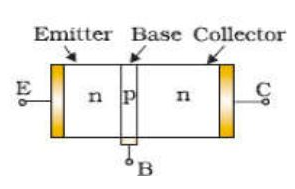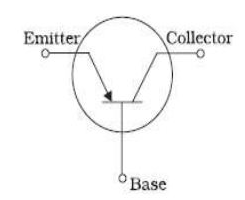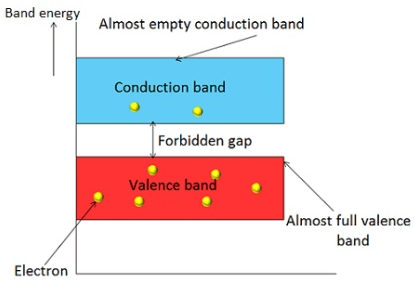Courses

# Test: Electronic Devices (CBSE Level)

## 30 Questions MCQ Test Exclusive Video Lectures of Class 12 Physics by Experts | Test: Electronic Devices (CBSE Level)

Description
This mock test of Test: Electronic Devices (CBSE Level) for Class 12 helps you for every Class 12 entrance exam. This contains 30 Multiple Choice Questions for Class 12 Test: Electronic Devices (CBSE Level) (mcq) to study with solutions a complete question bank. The solved questions answers in this Test: Electronic Devices (CBSE Level) quiz give you a good mix of easy questions and tough questions. Class 12 students definitely take this Test: Electronic Devices (CBSE Level) exercise for a better result in the exam. You can find other Test: Electronic Devices (CBSE Level) extra questions, long questions & short questions for Class 12 on EduRev as well by searching above.
QUESTION: 1

### Basic materials used in the present solid state electronic devices like diode, transistor, ICs, etc are

Solution:

Explanation:Semiconductors are materials that have electrical conductivity between conductors such as most metals and nonconductors or insulators like ceramics. Semiconductor material is used in the manufacturing of electrical components and used in electronic devices such as transistors and diodes.Semiconductors are the basic materials used in the present solid state electronic devices like diode, transistor, ICs etc

QUESTION: 2

### Depletion region (space charge) is formed because

Solution:

Explanation:The depletion region, also called depletion layer, depletion zone, junction region, space charge region or space charge layer, is an insulating region within a conductive, doped semiconductor material where the mobile charge carriers have been diffused away, or have been forced away by an electric field. The only elements left in the depletion region are ionized donor or acceptor impurities.The depletion region is so named because it is formed from a conducting region by removal of all free charge carriers, leaving none to carry a current.

QUESTION: 3

### The conductivity of P – type semiconductor is due to

Solution:
QUESTION: 4

In an N-P-N transistor, P-type crystal is

Solution:

Explanation:When the p-type crystal is grown between relatively wide sections of n-type crystals then the transistor is called NPN transistor.QUESTION: 5

Domestic power supply in India is

Solution:

Explanation:The supply of electric power to our homes from generating station is mainly in the form of alternating voltage in which there is change in the polarity of voltage on a regular cycle. AC can easily be transmitted to large distance also it can be step up or down easily using transformer. The voltage, expressed in terms of root mean square value, and frequency are two important parameters of AC.There is no common standard for these parameters and these vary among regions. The voltages are generally in the range of 100-240 V and the two commonly used frequencies are 50 and 60 hertz. In India,we use electricity at 230 volt and 50 hertz.

QUESTION: 6

In the case of metals the valence and conduction bands have

Solution:

Explanation:The materials can be classified by the energy gap between their valence band and the conduction band. The valence band is the band consisting of the valence electron, and the conduction band remains empty. Conduction takes place when an electron jumps from valence band to conduction band and the gap between these two bands is forbidden energy gap. Wider the gap between the valence and conduction bands, higher the energy it requires for shifting an electron from valence band to the conduction band.In the case of conductors, this energy gap is absent or in other words conduction band, and valence band overlaps each other. Thus, electron requires minimum energy to jump from valence band. The typical examples of conductors are Silver, Copper, and Aluminium.In insulators, this gap is vast. Therefore, it requires a significant amount of energy to shift an electron from valence to conduction band. Thus, insulators are poor conductors of electricity. Mica and Ceramic are the well-known examples of insulation material.Semiconductors, on the other hand, have an energy gap which is in between that of conductors and insulators. This gap is typically more or less 1 eV, and thus, one electron requires energy more than conductors but less than insulators for shifting valence band to conduction band.

QUESTION: 7

In a p-n junction, as the diffusion process continues the width of the depletion zone

Solution:
QUESTION: 8

An N-type Ge is obtained on doping the Ge- crystal with

Solution:

Explanation:The addition of pentavalent impurities such as antimony, arsenic or phosphorous contributes free electrons, greatly increasing the conductivity of the intrinsic semiconductor.

QUESTION: 9

A p- type semiconductor can be obtained by adding

Solution:

Explanation:In P-type doping, boron or gallium is the dopant. Boron and gallium each have only three outer electrons. When mixed into the silicon lattice, they form "holes" in the lattice where a silicon electron has nothing to bond to. The absence of an electron creates the effect of a positive charge, hence the name P-type.Holes can conduct current. A hole happily accepts an electron from a neighbor, moving the hole over a space. P-type silicon is a good conductor.

QUESTION: 10

In a semiconductor crystal, if current flows due to breakage of crystal bonds, then the semiconductor is called

Solution:

Explanation:In the case of an intrinsic semiconductor say Si where each Si is having 4 outermost electrons its crystal structure consists of making 4 covalent bonds with 4 neighbouring Si atoms. Each bond consists of two electrons.Now if one oi the bonds gets broken due to some reason collisions or high temperature then one electron gets free and it will be having sufficient energy to cross the band gap and be ready for conduction- So in intrinsic semiconductors current flows due to breakage of crystal bonds.

QUESTION: 11

Hole is a vacancy or lack of an electron and can travel through the semiconductor material. It can

Solution:

Explanation:Holes and electrons are the two types of charge carriers responsible for current in semiconductor materials.Holes in a metal or semiconductor crystal lattice can move through the lattice as electrons can, and act similarly to positively-charged particles. They play an important role in the operation of semiconductor devices such as transistors, diodes and integrated circuits. However they are not actually particles, but rather quasiparticles; they are different from the positron, which is the antiparticle of the electron.

QUESTION: 12

Which of the following is not a semiconductor?

Solution:

Explanation:Materials that have the resistance levels between those of a conductor and an insulator are referred to as semiconductors.They are quite common, found in almost all electronic devices. Good examples of semiconductor materials are germanium, selenium, and silicon.Radium is a chemical element with symbol Ra and atomic number 88. It is the sixth element in group 2 of the periodic table, also known as the alkaline earth metals.

QUESTION: 13

Majority current carriers in N – types are

Solution:

Explanation:The more abundant charge carriers are called majority carriers, which are primarily responsible for current transport in a piece of semiconductor. In n-type semiconductors they are electrons, while in p-type semiconductors they are holes.

QUESTION: 14

The depletion layer in the p-n junction is caused

Solution:
QUESTION: 15

When n –type of semiconductor is heated

Solution:

Explanation:Due to heating, when a free electron is produced then simultaneously a hole is also produced.

QUESTION: 16

In a pure, or intrinsic, semiconductor, valence band holes and conduction-band electrons are always present

Solution:

Explanation:An intrinsic semiconductor, also called an undoped semiconductor or i-type semiconductor, is a pure semiconductor without any significant dopant species present. The number of charge carriers is therefore determined by the properties of the material itself instead of the amount of impurities. In intrinsic semiconductors the number of excited electrons and the number of holes are equal: n = p.

QUESTION: 17

In a semiconductor, the forbidden energy gap between the valance band and the conduction band is of the order of

Solution:

Explanation:The material which has electrical conductivity between that of a conductor and an insulator is called as semiconductor. Silicon, germanium and graphite are some examples of semiconductors.In semiconductors, the forbidden gap between valence band and conduction band is very small. It has a forbidden gap of about 1 electron volt (eV).

QUESTION: 18

A solid having uppermost energy – band partially filled with electrons is called

Solution:
QUESTION: 19

Doping of semiconductor is the process of

Solution:

Explanation:Doping is the process of adding impurities to intrinsic semiconductors to alter their properties. Normally Trivalent and Pentavalent elements are used to dope Silicon and Germanium. When an intrinsic semiconductor is doped with Trivalent impurity it becomes a P-Type semiconductor.

QUESTION: 20

Hole is

Solution:

Concept of holes in the semiconductor:

1. When an electron is removed from a covalent bond, it leaves a vacancy behind. An electron from a neighbouring atom can move into this vacancy, leaving the neighbour with a vacancy. In this way the vacancy formed is called a hole (or cotter), and can travel through the material and serve as an additional current carriers.
2. A hole is considered as a seat of positive charge, having magnitude of charge equal to that of an electron.
3. Holes acts as a virtual charge, although there is no physical charge on it.
4. Effective mass of hole is more than an electron.
5. Mobility of hole is less than an electron.
QUESTION: 21

When a P- N junction is reversed biased, the flow of current across the junction is mainly due to

Solution:

When p-n junction is reverse biased, the flow of current is due to drifting of minority charge carriers across the junction.

QUESTION: 22

The main difference between conductors, semiconductors and insulators is because of

Solution:

Explanation:Forbidden gap plays a major role for determining the electrical conductivity of material. Based on the forbidden gap materials are classified in to three types, they are : Insulators : The forbidden gap between the valence band and conduction band is very large in insulators. The energy gap of insulator is approximately equal to 15 electron volts (eV).Conductors: In a conductor, valence band and conduction band overlap each other. Therefore, there is no forbidden gap in a conductor.Semiconductors: In semiconductors, the forbidden gap between valence band and conduction band is very small. It has a forbidden gap of about 1 electron volt (eV).

QUESTION: 23

The number of valence electrons in a good conductor is generally

Solution:

Explanation:The electron theory states that all matter is composed of atoms and the atoms are composed of smaller particles called protons, electrons, and neutrons. The electrons orbit the nucleus which contains the protons and neutrons. It is the valence electrons that we are most concerned with in electricity. These are the electrons which are easiest to break loose from their parent atom. Normally, conductors have three or less valence electrons; insulators have five or more valence electrons; and semiconductors usually have four valence electrons.

QUESTION: 24

In the middle of the depletion layer of a reverse biased p-n-junction, the

Solution:
QUESTION: 25

The output from a full wave rectifier is

Solution:
QUESTION: 26

Motion of hole is a convenient way of describing

Solution:

Explanation:The holes are just the abscence of a electron in a energy band. But its easier to describe the abscence of a electron as a single moving positive charge than it is to describe the motion of all the other electrons in the band.In a band you have N electrons. Remove one of those electrons(by for instance p-doping) and you have N-1 electrons left. Now you can either choose to describe this with the behavior of those N-1 electrons. Or you can choose to describe it as if there is one single hole moving around in the band.So mathematicly the holes behave just like a positivly charged electron.

QUESTION: 27

When the conductivity of a semiconductor is only due to breaking of the covalent bonds, the semiconductor is called

Solution:

Explanation:A pure semiconductor is called intrinsic semiconductor, e.g., silicon, germanium. The presence of the mobile charge carriers is the intrinsic property of the material. At room temperature, some covalent bonds are broken and electrons are made free. The absence of electron in the covalent bond form hole.The electrical conduction is by means of mobile electrons and holes. Hole act as positive charge, because it can attract an electron. If some other bond is broken and the electron thus freed fills this hole(vacancy), it seems as though the hole is moving.Actually an electron is travelling in opposite direction. In a pure(intrinsic) semiconductor, the number of holes is equal to the number of free electrons.

QUESTION: 28

Electrons are forbidden in a band (in a crystal) called the

Solution:

Explanation:A region of values of energy that electrons in an ideal crystal (without defects) cannot have. In semiconductors the forbidden band separating the valence band and the conduction band is usually considered. In this case the energy difference between the lower level (bottom) of the conduction band and the upper level (ceiling) of the valence band is called the width of the forbidden band.

QUESTION: 29

The difference in variation of resistance with temperature in a metal and semiconductor is due to

Solution:
QUESTION: 30

A piece of copper and another of germanium are cooled from room temperature to 80 K. The resistance of

Solution:

We know, a piece of copper is a metal while that of germanium is a semiconducting material. For metals resistance increases with increase in temperature. The semiconductor has a negative temperature coefficient of resistance. Hence, when it is cooled its resistance increases.